# How To Add Text Cells Together Into One Cell In Excel?

When we have two separate columns in an Excel sheet which are to be combined, you don't have to create a new column and enter the whole data again. In this tutorial, we will show you two different ways to combine text cells together into one cell. You can either used the Ampersand symbol (&) or the CONCAT function for this purpose.

We will take a simple example to demonstrate how it is done. We will take to columns that would contain the "first name" and "last name" of a set of people. Then, we will create a new column to show the full names of those people. Essentially we will combine the text of the first two columns to create a third column.

## Adding The Text Cells Together Using The Ampersand Symbol

The ampersand symbol is mostly used for combining two or more objects or columns.

### Step 1

Create an Excel sheet and insert data (first name and last name) under two columns, as shown here −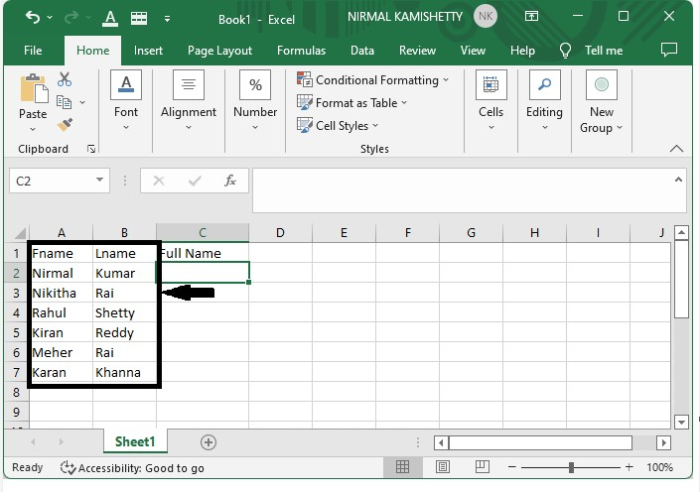### Step 2

Enter the formula (=address of first text box& " " &address of second box) in the formula box after clicking on the text cell where you want to display the result.

In our case it will be (=A2&" "&B2) in the formula box after clicking the text cell "C2".

Press the "Enter" button to get the first result.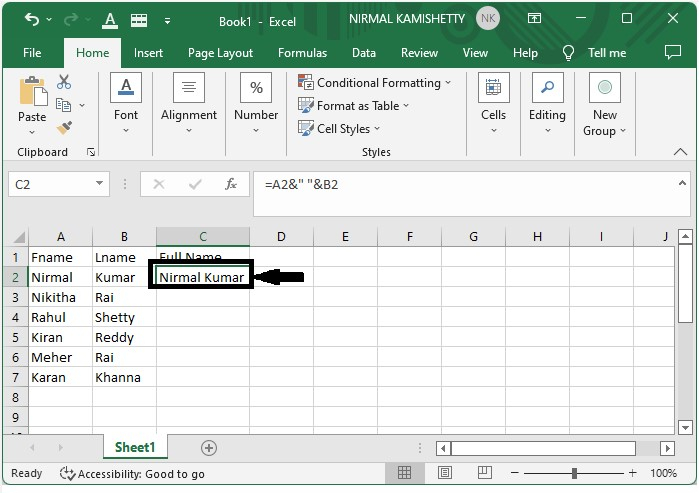### Step 3

After getting the first result, we can get all the other results just by dragging down from the right corner of the cell where the first result is present. The final output will appear as shown in the following screenshot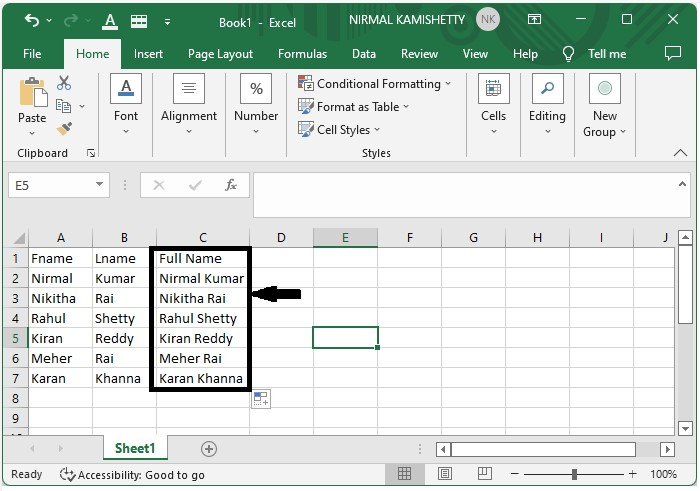## Adding The Text Cells Together Using The CONCAT Function

We can also use the CONCAT function instead of using the ampersand symbol. Let's find out how.

### Step 1

Let's use the same Excel sheet with two columns showing the first and last names of a set of people.### Step 2

In the formula box, enter the formula "=CONCAT (address of first data, address of second data)" after clicking the text cell where you want to display the result.

In our case, the formula will be "=CONCAT(A2, B2)". Press the "Enter" button to get the first result, as shown below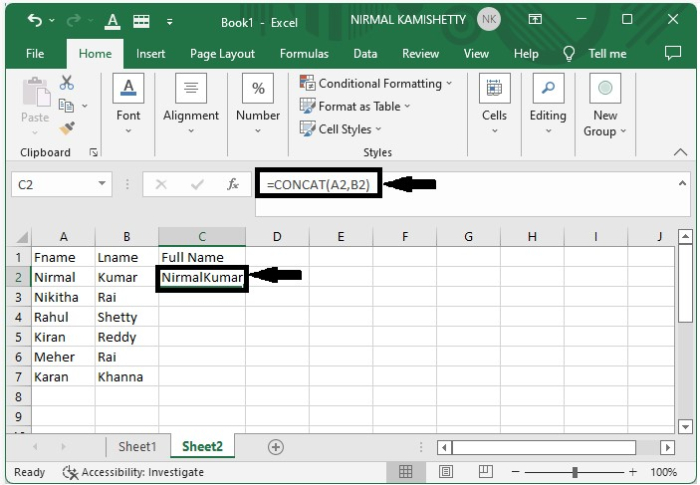### Step 3

To get the final result, drag from the right corner of the cell where the first result is present.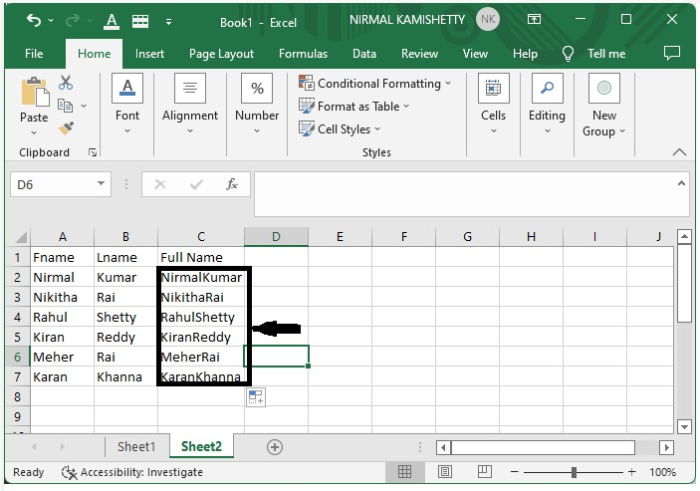## Conclusion

In this tutorial, we used a simple example to demonstrate how you can add text cells together into one cell in Excel in two different ways.